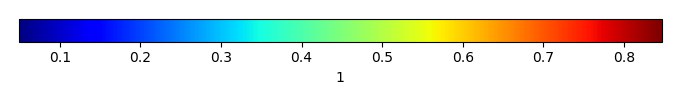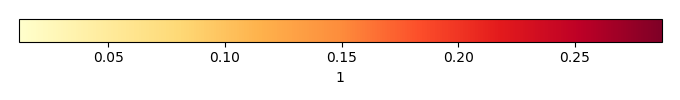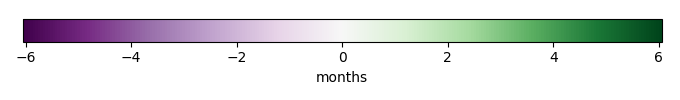# Mean State

Period Mean (original grids) 
Model Period Mean (intersection) 
Model Period Mean (complement) 
Benchmark Period Mean (intersection) 
Benchmark Period Mean (complement) 
Bias 
RMSE 
Phase Shift [months]
Bias Score 
RMSE Score 
Seasonal Cycle Score 
Spatial Distribution Score 
Interannual Variability Score 
Overall Score 
Benchmark [-] 0.138
CLM5PHSOFF [-] 0.158 0.157 0.00 0.143 0.0572 0.0141 0.0301 3.04 0.30 0.29 0.50 0.95 0.58 0.49
CLM5PHSON [-] 0.156 0.155 0.00 0.143 0.0572 0.0121 0.0287 3.03 0.32 0.31 0.50 0.94 0.55 0.49
Period Mean (original grids) 
Model Period Mean (intersection) 
Model Period Mean (complement) 
Benchmark Period Mean (intersection) 
Benchmark Period Mean (complement) 
Bias 
RMSE 
Phase Shift [months]
Bias Score 
RMSE Score 
Seasonal Cycle Score 
Spatial Distribution Score 
Interannual Variability Score 
Overall Score 
Benchmark [-] 0.181
CLM5PHSOFF [-] 0.190 0.192 0.00 0.182 0.0612 0.00871 0.0345 3.28 0.42 0.23 0.45 0.98 0.71 0.51
CLM5PHSON [-] 0.186 0.187 0.00 0.182 0.0612 0.00407 0.0324 2.99 0.43 0.26 0.51 0.97 0.65 0.51
Period Mean (original grids) 
Model Period Mean (intersection) 
Model Period Mean (complement) 
Benchmark Period Mean (intersection) 
Benchmark Period Mean (complement) 
Bias 
RMSE 
Phase Shift [months]
Bias Score 
RMSE Score 
Seasonal Cycle Score 
Spatial Distribution Score 
Interannual Variability Score 
Overall Score 
Benchmark [-] 0.142
CLM5PHSOFF [-] 0.158 0.158 0.00 0.146 0.0640 0.0117 0.0341 2.78 0.40 0.22 0.55 0.93 0.70 0.50
CLM5PHSON [-] 0.157 0.157 0.00 0.146 0.0640 0.0106 0.0316 2.50 0.40 0.27 0.60 0.91 0.63 0.51
Period Mean (original grids) 
Model Period Mean (intersection) 
Model Period Mean (complement) 
Benchmark Period Mean (intersection) 
Benchmark Period Mean (complement) 
Bias 
RMSE 
Phase Shift [months]
Bias Score 
RMSE Score 
Seasonal Cycle Score 
Spatial Distribution Score 
Interannual Variability Score 
Overall Score 
Benchmark [-] 0.368
CLM5PHSOFF [-] 0.347 0.347 0.00 0.370 0.336 -0.0238 0.136 1.29 0.75 0.59 0.85 0.98 0.75 0.75
CLM5PHSON [-] 0.322 0.322 0.00 0.370 0.336 -0.0485 0.125 1.11 0.72 0.61 0.89 0.98 0.72 0.76
Period Mean (original grids) 
Model Period Mean (intersection) 
Model Period Mean (complement) 
Benchmark Period Mean (intersection) 
Benchmark Period Mean (complement) 
Bias 
RMSE 
Phase Shift [months]
Bias Score 
RMSE Score 
Seasonal Cycle Score 
Spatial Distribution Score 
Interannual Variability Score 
Overall Score 
Benchmark [-] 0.0844
CLM5PHSOFF [-] 0.138 0.138 0.00 0.0928 0.0564 0.0451 0.0499 3.02 0.21 0.34 0.49 0.056 0.45 0.31
CLM5PHSON [-] 0.136 0.136 0.00 0.0928 0.0564 0.0432 0.0484 3.07 0.22 0.35 0.48 0.032 0.42 0.31
Period Mean (original grids) 
Model Period Mean (intersection) 
Model Period Mean (complement) 
Benchmark Period Mean (intersection) 
Benchmark Period Mean (complement) 
Bias 
RMSE 
Phase Shift [months]
Bias Score 
RMSE Score 
Seasonal Cycle Score 
Spatial Distribution Score 
Interannual Variability Score 
Overall Score 
Benchmark [-] 0.221
CLM5PHSOFF [-] 0.233 0.233 0.00 0.222 0.157 0.0105 0.132 1.16 0.76 0.50 0.88 0.96 0.74 0.72
CLM5PHSON [-] 0.200 0.199 0.00 0.222 0.157 -0.0233 0.0930 1.07 0.78 0.61 0.89 0.98 0.69 0.76
Period Mean (original grids) 
Model Period Mean (intersection) 
Model Period Mean (complement) 
Benchmark Period Mean (intersection) 
Benchmark Period Mean (complement) 
Bias 
RMSE 
Phase Shift [months]
Bias Score 
RMSE Score 
Seasonal Cycle Score 
Spatial Distribution Score 
Interannual Variability Score 
Overall Score 
Benchmark [-] 0.201
CLM5PHSOFF [-] 0.205 0.205 0.00 0.204 0.0927 0.000405 0.0647 1.08 0.67 0.48 0.84 0.94 0.71 0.69
CLM5PHSON [-] 0.201 0.202 0.00 0.204 0.0927 -0.00305 0.0621 1.09 0.68 0.49 0.84 0.94 0.70 0.69
Period Mean (original grids) 
Model Period Mean (intersection) 
Model Period Mean (complement) 
Benchmark Period Mean (intersection) 
Benchmark Period Mean (complement) 
Bias 
RMSE 
Phase Shift [months]
Bias Score 
RMSE Score 
Seasonal Cycle Score 
Spatial Distribution Score 
Interannual Variability Score 
Overall Score 
Benchmark [-] 0.138
CLM5PHSOFF [-] 0.159 0.159 0.00 0.144 0.0587 0.0147 0.0414 3.15 0.45 0.30 0.48 0.86 0.59 0.50
CLM5PHSON [-] 0.157 0.157 0.00 0.144 0.0587 0.0127 0.0399 3.25 0.47 0.32 0.45 0.85 0.52 0.49
Period Mean (original grids) 
Model Period Mean (intersection) 
Model Period Mean (complement) 
Benchmark Period Mean (intersection) 
Benchmark Period Mean (complement) 
Bias 
RMSE 
Phase Shift [months]
Bias Score 
RMSE Score 
Seasonal Cycle Score 
Spatial Distribution Score 
Interannual Variability Score 
Overall Score 
Benchmark [-] 0.367
CLM5PHSOFF [-] 0.347 0.344 0.00 0.366 0.392 -0.0213 0.119 1.50 0.79 0.60 0.83 0.98 0.76 0.76
CLM5PHSON [-] 0.339 0.337 0.00 0.366 0.392 -0.0292 0.111 1.40 0.79 0.62 0.85 0.98 0.76 0.77
Period Mean (original grids) 
Model Period Mean (intersection) 
Model Period Mean (complement) 
Benchmark Period Mean (intersection) 
Benchmark Period Mean (complement) 
Bias 
RMSE 
Phase Shift [months]
Bias Score 
RMSE Score 
Seasonal Cycle Score 
Spatial Distribution Score 
Interannual Variability Score 
Overall Score 
Benchmark [-] 0.153
CLM5PHSOFF [-] 0.170 0.170 0.00 0.160 0.0616 0.0105 0.0343 2.30 0.43 0.31 0.64 0.80 0.70 0.53
CLM5PHSON [-] 0.167 0.167 0.00 0.160 0.0616 0.00755 0.0333 2.40 0.44 0.33 0.62 0.78 0.68 0.53
Period Mean (original grids) 
Model Period Mean (intersection) 
Model Period Mean (complement) 
Benchmark Period Mean (intersection) 
Benchmark Period Mean (complement) 
Bias 
RMSE 
Phase Shift [months]
Bias Score 
RMSE Score 
Seasonal Cycle Score 
Spatial Distribution Score 
Interannual Variability Score 
Overall Score 
Benchmark [-] 0.146
CLM5PHSOFF [-] 0.168 0.168 0.00 0.153 0.0665 0.0154 0.0675 1.41 0.58 0.39 0.80 0.95 0.65 0.63
CLM5PHSON [-] 0.164 0.165 0.00 0.153 0.0665 0.0115 0.0634 1.43 0.58 0.41 0.80 0.91 0.67 0.63
Period Mean (original grids) 
Model Period Mean (intersection) 
Model Period Mean (complement) 
Benchmark Period Mean (intersection) 
Benchmark Period Mean (complement) 
Bias 
RMSE 
Phase Shift [months]
Bias Score 
RMSE Score 
Seasonal Cycle Score 
Spatial Distribution Score 
Interannual Variability Score 
Overall Score 
Benchmark [-] 0.147
CLM5PHSOFF [-] 0.274 0.274 0.00 0.262 0.0914 0.0118 0.0633 2.23 0.51 0.38 0.65 0.99 0.66 0.59
CLM5PHSON [-] 0.268 0.268 0.00 0.262 0.0914 0.00606 0.0584 2.21 0.51 0.40 0.65 0.99 0.64 0.60
Period Mean (original grids) 
Model Period Mean (intersection) 
Model Period Mean (complement) 
Benchmark Period Mean (intersection) 
Benchmark Period Mean (complement) 
Bias 
RMSE 
Phase Shift [months]
Bias Score 
RMSE Score 
Seasonal Cycle Score 
Spatial Distribution Score 
Interannual Variability Score 
Overall Score 
Benchmark [-] 0.284
CLM5PHSOFF [-] 0.314 0.314 0.00 0.293 0.0694 0.0211 0.0513 3.40 0.31 0.28 0.42 0.98 0.68 0.49
CLM5PHSON [-] 0.313 0.313 0.00 0.293 0.0694 0.0202 0.0516 3.39 0.31 0.28 0.42 0.98 0.67 0.49
Period Mean (original grids) 
Model Period Mean (intersection) 
Model Period Mean (complement) 
Benchmark Period Mean (intersection) 
Benchmark Period Mean (complement) 
Bias 
RMSE 
Phase Shift [months]
Bias Score 
RMSE Score 
Seasonal Cycle Score 
Spatial Distribution Score 
Interannual Variability Score 
Overall Score 
Benchmark [-] 0.399
CLM5PHSOFF [-] 0.373 0.373 0.00 0.400 0.375 -0.0269 0.118 1.27 0.78 0.62 0.84 0.99 0.74 0.77
CLM5PHSON [-] 0.363 0.363 0.00 0.400 0.375 -0.0369 0.118 1.19 0.77 0.63 0.85 0.99 0.72 0.77
Period Mean (original grids) 
Model Period Mean (intersection) 
Model Period Mean (complement) 
Benchmark Period Mean (intersection) 
Benchmark Period Mean (complement) 
Bias 
RMSE 
Phase Shift [months]
Bias Score 
RMSE Score 
Seasonal Cycle Score 
Spatial Distribution Score 
Interannual Variability Score 
Overall Score 
Benchmark [-] 0.113
CLM5PHSOFF [-] 0.150 0.150 0.00 0.125 0.0569 0.0243 0.0452 2.91 0.29 0.27 0.52 0.64 0.67 0.45
CLM5PHSON [-] 0.148 0.148 0.00 0.125 0.0569 0.0222 0.0432 3.04 0.30 0.30 0.49 0.62 0.60 0.43
Period Mean (original grids) 
Model Period Mean (intersection) 
Model Period Mean (complement) 
Benchmark Period Mean (intersection) 
Benchmark Period Mean (complement) 
Bias 
RMSE 
Phase Shift [months]
Bias Score 
RMSE Score 
Seasonal Cycle Score 
Spatial Distribution Score 
Interannual Variability Score 
Overall Score 
Benchmark [-] 0.154
CLM5PHSOFF [-] 0.166 0.165 0.00 0.159 0.0605 0.00665 0.0584 2.06 0.57 0.33 0.66 0.95 0.66 0.58
CLM5PHSON [-] 0.159 0.159 0.00 0.159 0.0605 0.000121 0.0454 2.03 0.58 0.38 0.66 0.89 0.68 0.59
Period Mean (original grids) 
Model Period Mean (intersection) 
Model Period Mean (complement) 
Benchmark Period Mean (intersection) 
Benchmark Period Mean (complement) 
Bias 
RMSE 
Phase Shift [months]
Bias Score 
RMSE Score 
Seasonal Cycle Score 
Spatial Distribution Score 
Interannual Variability Score 
Overall Score 
Benchmark [-] 0.193
CLM5PHSOFF [-] 0.200 0.200 0.00 0.197 0.0857 0.00411 0.116 0.803 0.69 0.51 0.93 0.97 0.68 0.72
CLM5PHSON [-] 0.174 0.175 0.00 0.197 0.0857 -0.0213 0.0864 0.732 0.73 0.59 0.93 0.98 0.64 0.74
Period Mean (original grids) 
Model Period Mean (intersection) 
Model Period Mean (complement) 
Benchmark Period Mean (intersection) 
Benchmark Period Mean (complement) 
Bias 
RMSE 
Phase Shift [months]
Bias Score 
RMSE Score 
Seasonal Cycle Score 
Spatial Distribution Score 
Interannual Variability Score 
Overall Score 
Benchmark [-] 0.192
CLM5PHSOFF [-] 0.186 0.186 0.00 0.194 0.0720 -0.00792 0.0616 1.35 0.66 0.41 0.80 0.99 0.70 0.66
CLM5PHSON [-] 0.177 0.177 0.00 0.194 0.0720 -0.0170 0.0587 1.51 0.62 0.43 0.77 0.98 0.71 0.66
Period Mean (original grids) 
Model Period Mean (intersection) 
Model Period Mean (complement) 
Benchmark Period Mean (intersection) 
Benchmark Period Mean (complement) 
Bias 
RMSE 
Phase Shift [months]
Bias Score 
RMSE Score 
Seasonal Cycle Score 
Spatial Distribution Score 
Interannual Variability Score 
Overall Score 
Benchmark [-] 0.134
CLM5PHSOFF [-] 0.137 0.137 0.00 0.136 0.0519 0.000703 0.0208 2.71 0.53 0.35 0.56 0.42 0.46 0.44
CLM5PHSON [-] 0.135 0.135 0.00 0.136 0.0519 -0.00112 0.0207 2.96 0.51 0.35 0.52 0.25 0.42 0.40
Period Mean (original grids) 
Model Period Mean (intersection) 
Model Period Mean (complement) 
Benchmark Period Mean (intersection) 
Benchmark Period Mean (complement) 
Bias 
RMSE 
Phase Shift [months]
Bias Score 
RMSE Score 
Seasonal Cycle Score 
Spatial Distribution Score 
Interannual Variability Score 
Overall Score 
Benchmark [-] 0.144
CLM5PHSOFF [-] 0.153 0.152 0.00 0.147 0.0557 0.00495 0.0255 3.02 0.48 0.20 0.49 0.81 0.66 0.48
CLM5PHSON [-] 0.149 0.149 0.00 0.147 0.0557 0.00174 0.0238 3.18 0.47 0.23 0.46 0.65 0.64 0.45
Period Mean (original grids) 
Model Period Mean (intersection) 
Model Period Mean (complement) 
Benchmark Period Mean (intersection) 
Benchmark Period Mean (complement) 
Bias 
RMSE 
Phase Shift [months]
Bias Score 
RMSE Score 
Seasonal Cycle Score 
Spatial Distribution Score 
Interannual Variability Score 
Overall Score 
Benchmark [-] 0.141
CLM5PHSOFF [-] 0.157 0.157 0.00 0.149 0.0759 0.00872 0.0478 2.13 0.44 0.32 0.67 0.90 0.66 0.55
CLM5PHSON [-] 0.159 0.160 0.00 0.149 0.0759 0.0109 0.0468 2.09 0.44 0.33 0.68 0.85 0.63 0.55

# Temporally integrated period mean

BENCHMARK MEANMODEL MEANBIASBIAS SCORERMSERMSE SCOREBENCHMARK INTERANNUAL VARIABILITYMODEL INTERANNUAL VARIABILITYINTERANNUAL VARIABILITY SCOREBENCHMARK MAX MONTHMODEL MAX MONTHDIFFERENCE IN MAX MONTHSEASONAL CYCLE SCORESPATIAL TAYLOR DIAGRAMMODEL COLORS# Spatially integrated regional mean

MODEL COLORSREGIONAL MEANANNUAL CYCLEMONTHLY ANOMALYANNUAL CYCLE# All Models

BenchmarkCLM5PHSOFFCLM5PHSON# Data Information

creation_date: Wed May 14 11:10:39 PDT 2014

source_file: This product is generated from monthly 1 degree CERES EBAF Radiation observations